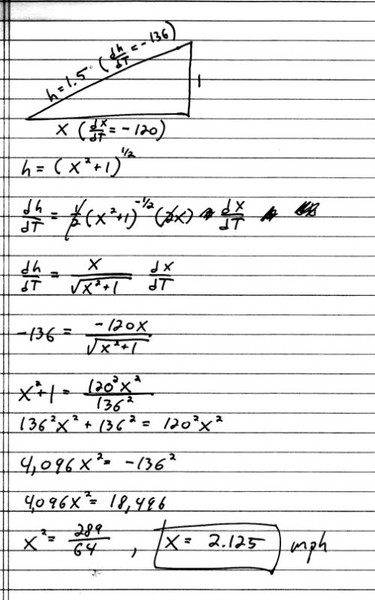# Related rates, check my answer pls

e^(i Pi)+1=0

## Homework Statement

A highway patrol plane is flying 1 mile above a long, straight road, with constant ground speed of 120 m.p.h. Using radar, the pilot detects a car whose distance from the plane is 1.5 miles and decreasing at a rate of 136 m.p.h. How fast is the car traveling along the highway?

## The Attempt at a Solution## The Attempt at a Solution

Homework Helper
It's wrong. The value of x in the related rates equation is not an unknown. x^2+1=1.5^2. It's easy to find. And 'x' is the distance, not a velocity. dx/dT is not -120. It's a combination of the plane's velocity with the unknown velocity of the car. That's what you want to solve for.

Last edited:
e^(i Pi)+1=0
So would you say that (velocity of car)$\frac{dc}{dt} = 120 + \frac{dx}{dt}$

so that

$\frac{dh}{dt}=\frac{x}{\sqrt{x^2+1}}\frac{dx}{dt}$

where

$\frac{dx}{dt} = \frac{dc}{dt} - 120$

$\frac{dh}{dt}= -136$

and

x=$\sqrt{1.25}$

so that $\frac{dc}{dt} \approx -62.46 \approx 62.46 mph$

Last edited:
Homework Helper
So would you say that (velocity of car)$\frac{dc}{dt} = 120 + \frac{dx}{dt}$

so that

$\frac{dh}{dt}=\frac{x}{\sqrt{x^2+1}}\frac{dx}{dt}$

where

$\frac{dx}{dt} = \frac{dc}{dt} - 120$

$\frac{dh}{dt}= -136$

and

x=$\sqrt{1.25}$

so that $\frac{dc}{dt} \approx -62.46 \approx 62.46 mph$

Yes, I think that's more like it.